Courses

# Calculus (Part - 1) - Civil Engineering Civil Engineering (CE) Notes | EduRev

## Topic wise GATE Past Year Papers for Civil Engineering

Created by: Gate Gurus

## Civil Engineering (CE) : Calculus (Part - 1) - Civil Engineering Civil Engineering (CE) Notes | EduRev

The document Calculus (Part - 1) - Civil Engineering Civil Engineering (CE) Notes | EduRev is a part of the Civil Engineering (CE) Course Topic wise GATE Past Year Papers for Civil Engineering.
All you need of Civil Engineering (CE) at this link: Civil Engineering (CE)

Question 1: What is curl of the vector field 2x2yi + 5z2j - 4yzk?    [2019 : 1 Mark, Set-ll]
(a) -14zi-2x2k
(b) 6zi + 4x2j - 2x2k
(c) - 14zi + 6yj + 2x2k
(d) 6zi - 8xyj + 2x2yk
(a)
Solution: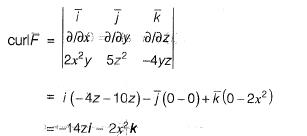Question 2: Euclidean norm (length) of the vector [4 -2 -6]r is    [2019 : 1 Mark, Set-ll]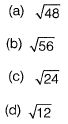Solution: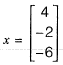Euclidean norm length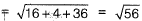Question 3: The following inequality is true for all x close to 0.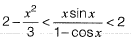What is tha value of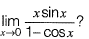[2019 : 1 Mark, Set-ll]
(a) 1
(b) 0
(c) 1/2
(d) 2
Solution: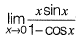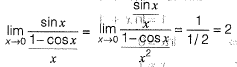Question 4: Consider the functions: x = y In φ and y = φ In y. which one of the following the correct expression for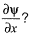[2019 : 2 Mark, Set-l]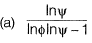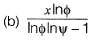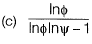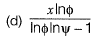Solution: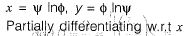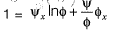......(i)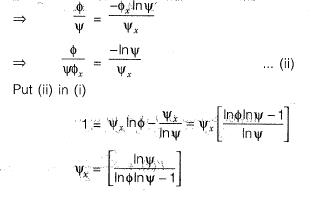Question 5: Which one of the following is NOT a correct statement?    [2019 : 2 Marks, Set-I]
(a) The function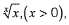has the global minima at x = e
(b) The functionhas the global maxima at x = a
(c) The function x3 has neither global minima nor global maxima
(d) The function |x| has the global minima at x = 0
Solution: Let y = x1/x
log y = logx/x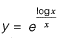y maximum (or) minimum when,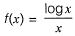is maximum (or) minimum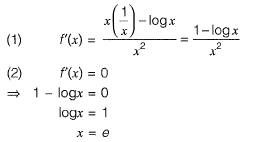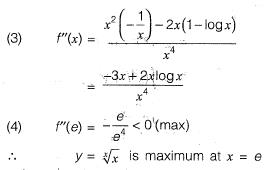Question 6: For a small value of h, the Taylor series expansion for f(x +h) is [2019 : 1 Mark, Set-I]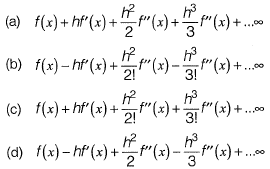Solution: Taylor series of f (x + h) at x.
f(x + h) = f(x) + (x + h - x)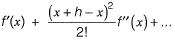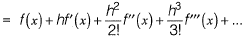Question 7: Which of the following is correct?    [2019 : 1 Mark, Set-I]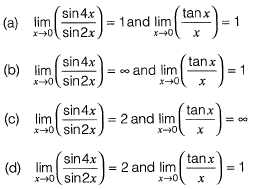Solution: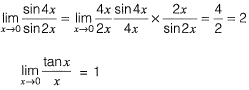Question 8: The value (up to two decimal places) of a line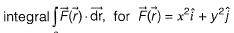along C which is a straight line joining (0, 0) to (1, 1) is _____.    [2018 : 2 Marks, Set-II]
Solution: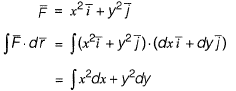(0, 0) to (1, 1) line is y = x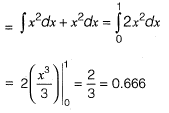Question 9: The value of the integral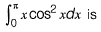[2018 : 2 Marks, Set-I]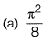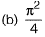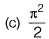(d) ∏2
Solution: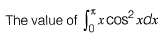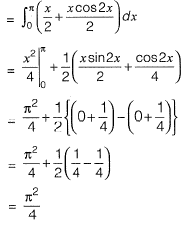Question 10: At the point x = 0, the function f(x) = x3 has    [2018 : 1 Marks, Set-I]
(a) local maximum
(b) local minimum
(c) both local maximum and minimum
(d) neither local maximum nor local minimum
Solution: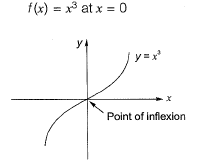Question 11: Consider the following definite integral: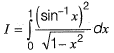The value of the integral is    [2017 : 2 Marks, Set-II]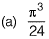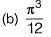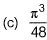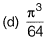Solution: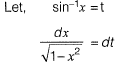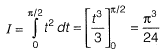Question 12: The tangent to the curve represented by y = x In x is required to have 45° inclination with the x-axis. The coordinates of the tangent point would be    [2017 : 2 Marks, Set-II]
(a) (1,0)
(b) (0,1)
(c) (1,1)
(d)Solution: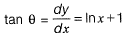tan 45° = In x + 1
1 = lnx + 1
⇒ Inx = 0
∴ x = 1

Putting x = 1 in the eq. of curve, we get y = 0.

Question 13:  The divergence of the vector field V = x2i + 2y3j + z4k at x = 1, y = 2, z = 3 is _________.    [2017 : 1 Mark, Set-II]
Solution: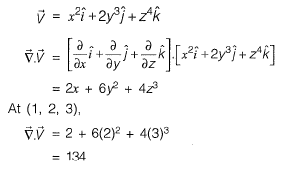Question 14: Let w= f(x, y), where x and yare functions of t. Then, according to the chain rule dw/dt    [2017 : 1 Mark, Set-II]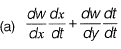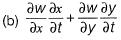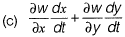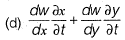Solution: W = f(x, y)
By Chain rule,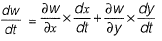Question 15: Let x be a continuous variable defined over the interval (-∞, ∞), and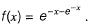The integral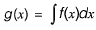is equal to    [2017 : 1 Mark, Set-I]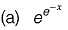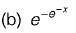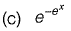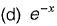Solution: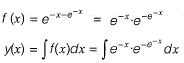Let  e-x = t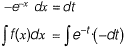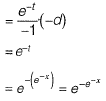Offer running on EduRev: Apply code STAYHOME200 to get INR 200 off on our premium plan EduRev Infinity!

,

,

,

,

,

,

,

,

,

,

,

,

,

,

,

,

,

,

,

,

,

;